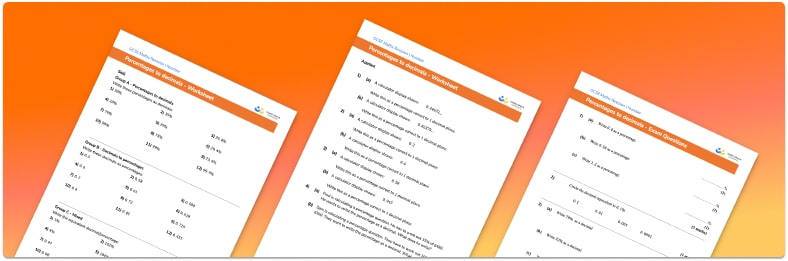# Percent To Decimal Worksheet• Section 1 of the percent to decimal worksheet contains 36 skills-based percent to decimal questions, in 3 groups to support differentiation
• Section 2 contains 4 applied percent to decimal questions with a mix of word problems and deeper problem solving questions
• Section 3 contains 4 foundation and higher level GCSE exam style percent to decimal questions
• Answer keys and a mark scheme for all percent to decimal questions are provided
• Questions follow variation theory with plenty of opportunities for students to work independently at their own level
• All math worksheets and questions are created by fully qualified expert secondary maths teachers
• Suitable for GCSE maths revision for AQA, OCR and Edexcel exam boards

• This field is for validation purposes and should be left unchanged.

You can unsubscribe at any time (each email we send will contain an easy way to unsubscribe). To find out more about how we use your data, see our privacy policy.

### Percent to decimal at a glance

Percentages and decimals are different formats for representing a part of a whole.

To convert from decimals to percentages we need to multiply the decimal by 100. Remember in order to multiply by 100 each number moves two place values to the left whilst the decimal point remains in the same place. An alternative way to think of this is that the percentage is the number of hundredths. Conversely, to convert from percentages to decimals we do the opposite and divide by 100.

To convert fractions to a decimal number, we could first convert them to a percentage by finding an equivalent fraction with a denominator of 100; the numerator would then be the percentage. Once we have the percentage, we can easily convert to a decimal by dividing by 100. We could alternatively find the decimal by dividing the numerator by the denominator and then multiply by 100 to find the percentage.

Looking forward, students can then progress to additional number worksheets, for example aor aFor more teaching and learning support on Number our GCSE maths lessons provide step by step support for all GCSE maths concepts.

## Do you have KS4 students who need more focused attention to succeed at GCSE?There will be students in your class who require individual attention to help them succeed in their maths GCSEs. In a class of 30, it’s not always easy to provide.

Help your students feel confident with exam-style questions and the strategies they’ll need to answer them correctly with our dedicated GCSE maths revision programme.

Lessons are selected to provide support where each student needs it most, and specially-trained GCSE maths tutors adapt the pitch and pace of each lesson. This ensures a personalised revision programme that raises grades and boosts confidence.

Find out more